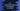# 3 different C++ programs to find the last index of a character in a string## How to find the last index of a character in a string in C++:

In this post, we will learn how to find the last index of a specific character in a given string in C++. We will write one C++ program that will take the string as input from the user and also the character to find. It will then scan the string to find the last index of that character in that string.

This problem can be solved in different ways. In this post, I will show you 3 different ways to solve this.

### Method 1: By iterating through the characters one by one from left to right:

This is the basic way to solve this problem. We will iterate through the characters of the string one by one and compare each character if it is equal to the provided character or not. If yes, we will print the index.

#### C++ program:

Below is the complete program:

``````#include<iostream>
#include<string>

using namespace std;

int findLastIndex(string givenStr, char c){
int index = -1;
int size = givenStr.length();

for(int i = 0; i<size; i++){
if(givenStr[i] == c){
index = i;
}
}
return index;
}

int main(){
string givenString;
char ch;

cout<<"Enter a string :"<<endl;
getline(cin, givenString);

cout<<"Enter a character to find the last index :"<<endl;
cin>>ch;

int index = findLastIndex(givenString, ch);
if(index == -1){
}else{
cout<<"Last index : "<<index;
}

}``````

Here,

• findLastIndex method is used to find the last index of a character in a string. It takes one string and one character. It scans all characters of the string one by one from left to right. If it finds the given character in any index, it assigns that value to the variable index. If the character is found multiple times, index gets updated on each time and at the end of this function, it holds the last position of that character. At end, we are returning index. If the character is not found, it returns -1.
• In the program, we are reading one string as user input to the variable givenString and to the character ch.
• We are calling findLastIndex to get the last index of the user input character and based on its value, we are printing one message.

#### Sample output:

``````Enter a string :
hello world
Enter a character to find the last index :
o
Last index : 7

Enter a string :
hello world
Enter a character to find the last index :
x

The time complexity for this method is O(n)

### Method 2: By iterating through the characters one by one from end to start:

We are trying to find out the last index position of a character. But we are iterating through all the characters of the string. If we have a large string e.g. a string with thousand of characters, it will take time to scan all characters in the string.

But, we can also improve this process by iterating through the characters from the end of the string. So, if we find that character at any position while iterating, we can return its index, because this is the last index for that character.

If we modify the above program, it will look as like below:

``````#include<iostream>
#include<string>

using namespace std;

int findLastIndex(string givenStr, char c){
int size = givenStr.length();

for(int i = size - 1; i>=0; i--){
if(givenStr[i] == c){
return i;
}
}
return -1;
}

int main(){
string givenString;
char ch;

cout<<"Enter a string :"<<endl;
getline(cin, givenString);

cout<<"Enter a character to find the last index :"<<endl;
cin>>ch;

int index = findLastIndex(givenString, ch);
if(index == -1){
}else{
cout<<"Last index : "<<index;
}

}``````

Here,

• I changed only the findLastIndex method. It is iterating the characters of the string from end to start and if a character is found equal to the given value, it is returning that index. Else, it returns -1.

#### Sample Output:

``````hello world
Enter a character to find the last index :
d
Last index : 10``````

With this method, we don’t have to iterate the whole string.

The time complexity for this method is O(n).

### Method 3: Using string::find_last_of :

find_last_of is a member function of the string class and we can use this function to find the last index of a character in a string. Below is the definition of this function:

``find_last_of(char c)``

To use this function, we need to include header file. It take one parameter i.e. the character to find in the string. And, it returns the last index of the character in the string. Else, it returns string::npos.

Below is the complete C++ program:

``````#include<iostream>
#include<string>

using namespace std;

int main(){
string givenString;
char ch;

cout<<"Enter a string :"<<endl;
getline(cin, givenString);

cout<<"Enter a character to find the last index :"<<endl;
cin>>ch;

int index = givenString.find_last_of(ch);
if(index == string::npos){
}else{
cout<<"Last index : "<<index;
}

}``````

It will give similar output as the above programs :

``````Enter a string :
hello world
Enter a character to find the last index :
o
Last index : 7

Enter a string :
hello world
Enter a character to find the last index :
x